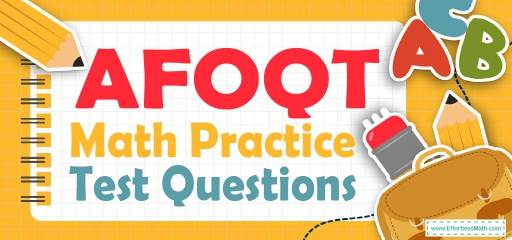# AFOQT Math Practice Test QuestionsPreparing for the AFOQT Arithmetic and Mathematics Knowledge test? Try these free AFOQT Math Practice questions. Reviewing practice questions is the best way to brush up on your Math skills. Here, we walk you through solving 10 common AFOQT Math practice problems covering the most important math concepts on the AFOQT Math test.

These AFOQT Math practice questions are designed to be similar to those found on the real AFOQT Math test. They will assess your level of preparation and will give you a better idea of what to study for your exam.

## 10 Sample AFOQT Math Practice Questions

1- A card is drawn at random from a standard 52–card deck, what is the probability that the card is of Hearts? (The deck includes 13 of each suit clubs, diamonds, hearts, and spades)

A. $$\frac{1}{3}$$

B. $$\frac{1}{6}$$

C. $$\frac{1}{52}$$

D. $$\frac{1}{4}$$

2- $$(5x + 5) (2x + 6) =$$ ?

A. $$5x + 6$$

B. $$10x^2 + 40x + 30$$

C. $$5x + 5x + 30$$

D. $$5x^2 + 5$$

3- The mean of 50 test scores was calculated as 88. But, it turned out that one of the scores was misread as 94 but it was 69. What is the mean?

A. 85

B. 87

C. 87.5

D. 88.5

4- $$5 (a – 6) = 22$$, what is the value of $$a$$?

A. 2.4

B. 10.4

C. 7

D. 11

5- If $$3^{24}=3^8× 3^x$$, what is the value of $$x$$?

A. 2

B. 1.5

C. 3

D. 16

6- Jason is 9 miles ahead of Joe running at 5.5 miles per hour and Joe is running at the speed of 7 miles per hour. How long does it take Joe to catch Jason?

A. 3 hours

B. 4 hours

C. 6 hours

D. 8 hours

7- 55 students took an exam and 11 of them failed. What percent of the students passed the exam?

A. $$40\%$$

B. $$60\%$$

C. $$80\%$$

D. $$20\%$$

8- Factor this expression: $$x^2 + 5 − 6$$

A. $$x^2(5 + 6)$$

B. $$x(x + 5 – 6)$$

C. $$(x + 6)(x – 1)$$

D. $$(x + 6)(x – 6)$$

9- Find the slope of the line running through the points $$(6, 7)$$ and $$(5, 3)$$.

A. $$\frac{1}{4}$$

B. 4

C. $$-4$$

D. $$-\frac{1}{4}$$

10- What is the missing term in the given sequence?
$$2, 3, 5, 8, 12, 17, 23, _, 38$$

A. 30

B. 28

C. 27

D. 25

## Best AFOQT Math Prep Resource for 2022

1- D
The probability of choosing a Hearts is $$\frac{13}{52}=\frac{1}{4}$$

2- B
Use FOIL (first, out, in, last) method.
$$(5x + 5) (2x + 6) = 10x^2 + 30x + 10x + 30 = 10x^2 + 40x + 30$$

3- C
$$average (mean) =\frac{(sum \space of \space terms)}{(number \space of \space terms)} {\Rightarrow} 88 = \frac{(sum \space of \space terms)}{50} {\Rightarrow} sum = 88 {\times} 50 = 4400$$
The difference of 94 and 69 is 25. Therefore, 25 should be subtracted from the sum.
$$4400 – 25 = 4375$$
$$mean = \frac{(sum of terms)}{(number of terms)} ⇒ mean = \frac{(4375)}{50}= 87.5$$

4- B
$$5(a–6)=22 ⇒ 5a-30=22 ⇒5a=22+30=52$$
$$⇒ 5a=52⇒a=\frac{52}{5}= 10.4$$

5- D
Use exponent multiplication rule:
$$x^a . x^b = x^{a + b}$$
Then:
$$3^{24}=3^8× 3^x=3^{8+x}$$
$$24 = 8 + x ⇒x=24-8=16$$

6- C
The distance between Jason and Joe is 9 miles. Jason running at 5.5 miles per hour and Joe is running at the speed of 7 miles per hour. Therefore, every hour the distance is 1.5 miles less. $$9 \div 1.5 = 6$$

7- C
The failing rate is 11 out of $$55 = \frac{11}{55}$$
Change the fraction to percent:
$$\frac{11}{55} {\times} 100\%=20\%$$
20 percent of students failed. Therefore, 80 percent of students passed the exam.

8- C
To factor the expression $$x^2 + 5 – 6$$, we need to find two numbers whose sum is 5 and their product is $$-6$$.
Those numbers are 6 and $$-1$$. Then:
$$x^2+5-6=(x+6)(x-1)$$

9- B
Slope of a line:$$\frac{y_2- y_1}{x_2 – x_1}=\frac{rise}{run}$$
$$\frac{y_2- y_1}{x_2 – x_1}=\frac{3- 7}{5 – 6}=\frac{-4}{-1}= 4$$

10- A
The difference of 2 and 3 is 1, 3 and 5 is 2, 5 and 8 is 3, 8 and 12 is 4, 12 and 17 is 5, 17 and 23 is 6, 23 and next number should be 7. The number is $$23 + 7 = 30$$

Looking for the best resource to help you succeed on the AFOQT Math test?

## More from Effortless Math for AFOQT Test …

### Do you have any questions about preparing for the AFOQT Math test?

Check out our How to Prepare for the AFOQT Math Test?

### Need AFOQT Math worksheets?

Have a look at our comprehensive AFOQT Math Worksheets to help you practice all AFOQT Math concepts and prepare for the AFOQT Math test.

Also, you can get more information about AFOQT test tips in the following post: AFOQT Math-Test Day Tips.

## Have any questions about the AFOQT Test?

### What people say about "AFOQT Math Practice Test Questions - Effortless Math: We Help Students Learn to LOVE Mathematics"?

No one replied yet.

X
52% OFF

Limited time only!

Save Over 52%

SAVE $40 It was$76.99 now it is \$36.99